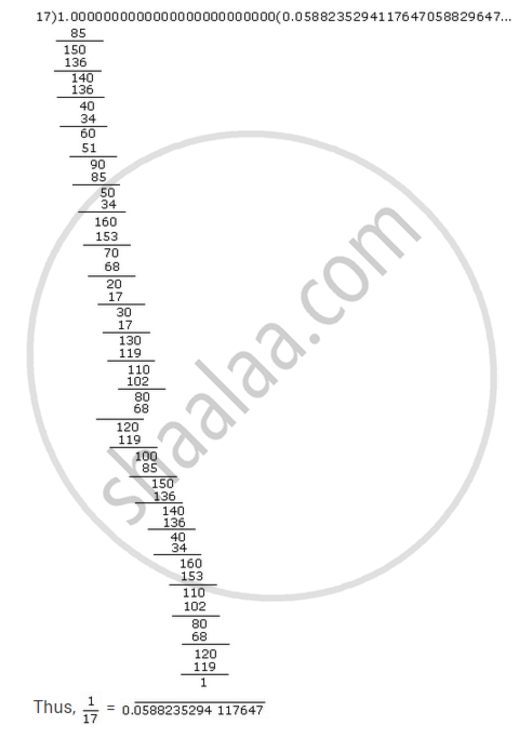# What can the maximum number of digits be in the repeating block of digits in the decimal expansion of 1/17? Perform the division to check your answer. - Mathematics

What can the maximum number of digits be in the repeating block of digits in the decimal expansion of 1/17? Perform the division to check your answer.

#### Solution

The maximum number of digits in the repeating block of digits in the decimal expansion of 1/17 can be 16.By long Division, the number of digits in the repeating block of digits in the decimal expansion of 1/17 = 16.

Concept: Real Numbers and Their Decimal Expansions
Is there an error in this question or solution?
Chapter 1: Number Systems - Exercise 1.3 [Page 14]

#### APPEARS IN

NCERT Class 9 Maths
Chapter 1 Number Systems
Exercise 1.3 | Q 5 | Page 14

Share